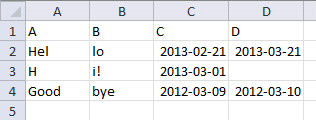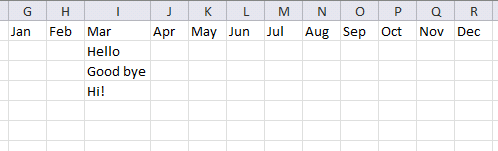Author: Oscar Cronquist Article last updated on February 25, 2013

I've a query in case of two columns of dates and two columns of data.
Calling columns A and B as data(text), C and D as Dates(dd/mm/yy),
IF column D's date is not empty and matches to the range, concatenate A & B and paste in in different sheet,
ELSE column C's date(column C will always have a date unlike column D) should be taken for range calculation and then concatenate A & B cells and paste in a cell.
Please do help in regards to this query

The two pictures below are from the same worksheet.

DataSortingArray formula in cell G1:

=IFERROR(IFERROR(INDEX(\$A\$2:\$A\$4&\$B\$2:\$B\$4, SMALL(IF((\$D\$2:\$D\$4<>"")*(TEXT(\$D\$2:\$D\$4, "mmm")=G\$1), MATCH(ROW(\$D\$2:\$D\$4), ROW(\$D\$2:\$D\$4)), ""), ROW(A1))), INDEX(\$A\$2:\$A\$4&\$B\$2:\$B\$4, SMALL(IF((\$D\$2:\$D\$4="")*(TEXT(\$C\$2:\$C\$4, "mmm")=G\$1), MATCH(ROW(\$D\$2:\$D\$4), ROW(\$D\$2:\$D\$4)), ""), ROW(A1)-SUMPRODUCT((\$D\$2:\$D\$4<>"")*(TEXT(\$D\$2:\$D\$4, "mmm")=G\$1))))), "")

How to enter an array formula

1. Select cell G1
2. Click in formula bar
3. Paste array formula to formula bar4. Press and hold CTRL + SHIFT
5. Press Enter

How to copy the array formula

1. Copy cell G2
2. Paste to cell range H2:R2
3. Copy cell range G2:R2
4. Paste to cell range G3:R10